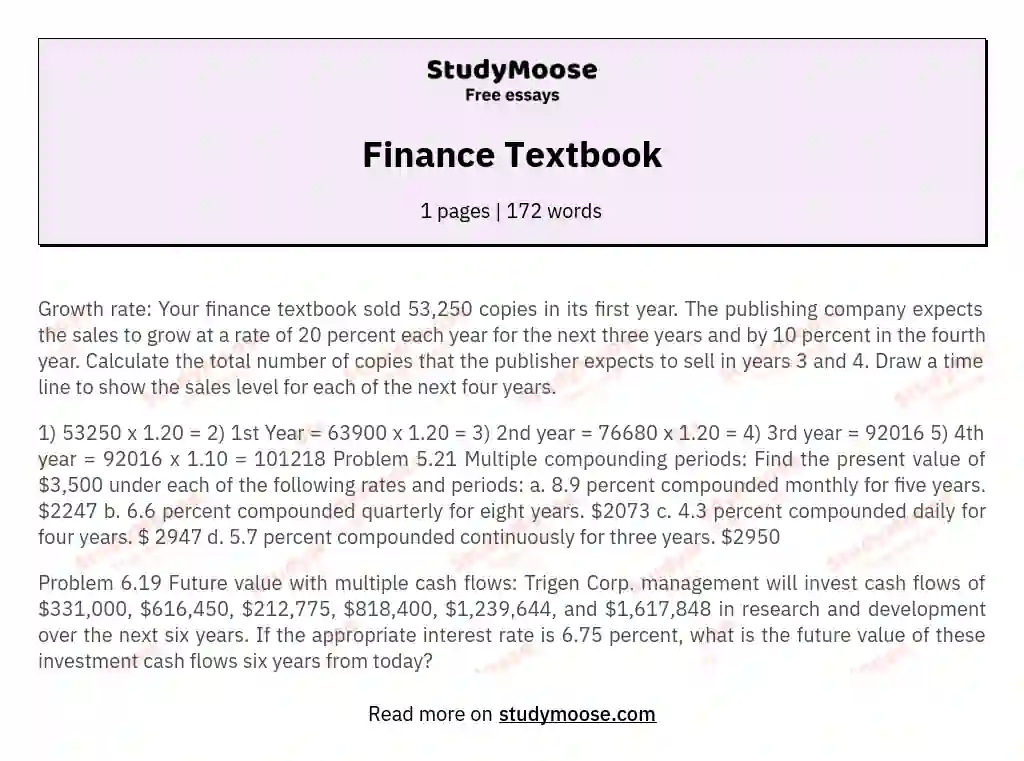# Finance Textbook

Categories: Finance

Growth rate: Your finance textbook sold 53,250 copies in its first year. The publishing company expects the sales to grow at a rate of 20 percent each year for the next three years and by 10 percent in the fourth year. Calculate the total number of copies that the publisher expects to sell in years 3 and 4. Draw a time line to show the sales level for each of the next four years.

1) 53250 x 1.20 =
2) 1st Year = 63900 x 1.20 =
3) 2nd year = 76680 x 1.20 =
4) 3rd year = 92016
5) 4th year = 92016 x 1.

10 = 101218
Problem 5.21
Multiple compounding periods: Find the present value of \$3,500 under each of the following rates and periods:
a. 8.9 percent compounded monthly for five years. \$2247
b. 6.6 percent compounded quarterly for eight years. \$2073
c. 4.3 percent compounded daily for four years. \$ 2947
d. 5.7 percent compounded continuously for three years. \$2950

Problem 6.19
Future value with multiple cash flows: Trigen Corp. management will invest cash flows of \$331,000, \$616,450, \$212,775, \$818,400, \$1,239,644, and \$1,617,848 in research and development over the next six years. If the appropriate interest rate is 6.75 percent, what is the future value of these investment cash flows six years from today?